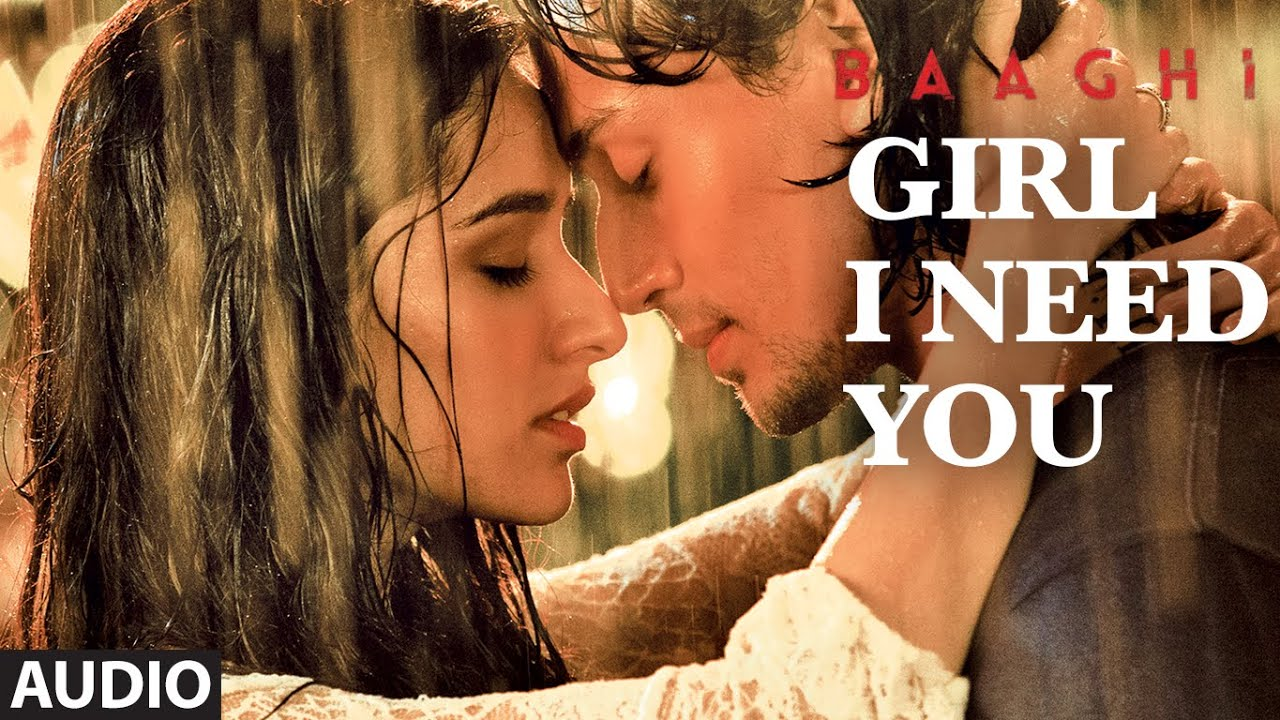# Girl I Need You Piano Notes Full – Baaghi

## Girl I Need You Piano Notes Full , Keyboard Notations , Casio NotationsSong : Girl I Need You
Singer : EET BROS,FEAT. ARIJIT SINGH,RAP: ROACH KILLA,KHUSHBOO GREWAL
Lyric : Sanjeev Chaturvedi
Casting : Tiger Shroff, Shraddha Kapoor
Music on : T-Series

Tujhpe meri hai daavedariyan
A(x3)+C+G+E+E+D+E+G+G
Tujhse hai dil ki saajhedariyan , saajhedariyan
A(x3)+C+G+E+E+D+E+F+E+D+C , C(x3)+D+E+F+E
Tujhpe meri hai daavedariyan
A(x3)+C+G+E+E+D+E+G+G
Tujhse hai dil ki saajhedariyan
A(x3)+C+G+E+E+D+E+F+E

Teri bin saansein loon to jee na sakoon
G+A+A+C(x5)+B+B+D+C+B+A+G
Ab tu hi bata kya main karoon
G+A+A+C(x5)+B+B+D+C+B+A+G
Kya karoon, kya karoon…
G+A+A , C+B+G+F+E+D+E

Girl I need you
E+D+E+G
Girl I need you
D+D+D+C
Girl I need you
E+D+E+G
Never leave you
A+A+C+G

Girl I need you
G+F+E+D
Girl I need you
D+C+D+C
Girl I need you
F+E+D+E
Never leave you
C+C+D+C

Subah se shaam tak lamhe
E+E+D+E+E+D+E+G

Baahon mein teri guzaarun
D(x4)+E+A+A+C
Chehra tera saamne rakh ke
E+E+D+E+E+D+E+G
Iss dil mein aaj utaarun
D(x4)+E+A+A+C

Hmmm hu , hmmm hu
E+D , D+D+E+C

Hmmm HU , Hmmmmhuhu
E+D , G+F+G+F+E

Aankhon ka nasha gulabi
E+E+D+E+E+D+E+G
Ab raha na jaaye zara bhi
D(x4)+E+A+A+C
Tu de permission pee loon
E+E+D+E+E+D+E+G
Tere naam ka banu sharabi
G(x4)+A+A
Utre na teri khumari
A(x4)+C+G+G+E
Bhulun main duniya saari
A(x4)+C+G+E

Oh girl every time I see you
A(x3)+G+A+G+A
G+G+F+D+F+E+E

Girl I need you
E+D+E+G
Girl I need you
D+D+D+C
Girl I need you
E+D+E+G
Never leave you
A+A+C+G

Girl I need you
G+F+E+D
Girl I need you
D+C+D+C
Girl I need you
F+E+D+E
Never leave you
C+C+D+C

Pehli dafa..
D+E+D+E
Aankhon se hai ye neendein khafa
G+G+F+E+C+D+E+D+E
Ke teri ore chal pada
G+G+F+E+C+E+F+E+F
Ye dil ka raasta (x2)
F+F+A+A+G+F+G

G+A+A+C(x5)+B+B+D+C+B+A+G
Ab judne lagi kya main karoon
G+A+A+D+D+C+C+B+D+C+B+A+G
Kya karoon, kya karoon
G+A+A , C+B+G+F+E+D+E

Girl I need you
E+D+E+G
Girl I need you
D+D+D+C
Girl I need you
E+D+E+G
Never leave you
A+A+C+G

Girl I need you
G+F+E+D
Girl I need you
D+C+D+C
Girl I need you
F+E+D+E
Never leave you
C+C+D+C

Lamhe maine tere liye hain rakh diye
C+B+G+C+B+G+D(x3)+E+D+B+D+D+C+C
Dil ye tujhko de diya hai bin kuch liye (x2)
C+B+G+C+B+G+D(x3)+E+D+B+D+D+C+C

Teri nazron ki taraf nazrein
G+A+A+C(x5)+B+B+D+C+B+A+G
Ab mudne lagi kya main karoon
G+A+A+D+D+C+C+B+D+C+B+A+G
Kya karoon, kya karoon
G+A+A , C+B+G+F+E+D+E

Girl I need you
E+D+E+G
Girl I need you
D+D+D+C
Girl I need you
E+D+E+G
Never leave you
A+A+C+G

Girl I need you
G+F+E+D
Girl I need you
D+C+D+C
Girl I need you
F+E+D+E
Never leave you
C+C+D+C

Also See :

For Buying Full Notes Send Email – [email protected]

if you have liked this post please share this post on social networks it will help us reaching more people arround the world you can share Bol Do Na Zara Piano Notes Full by using below social networks buttons.